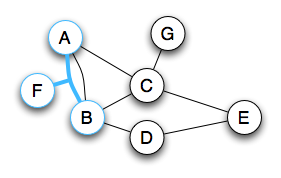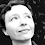## 5.30.2011

### Hypergraphs and Layer-3 Topology

In 2009, I posted some code to document a network's topology from a layer-3 perspective. Since that time, the quick-and-dirty BASH scripts have slowly been replaced, and a database and web interface have been added, making for a surprisingly robust tool that I may, one day, package and release for public consumption and scrutiny. But, while being able to draw layer-3 topologies is very nice, it is the addition of a centralized database, filled with topology data, that is the catalyst for continued exploration.

In January of this year, I worked out a simple algorithm to tackle the following problem:

Given an undirected graph G = (VE), find all simple paths having terminal vertices vs and vt for all vs ∈ V.

Some time has elapsed, but I was finally able to put code to my paperbound scrawl. Fortunately, this problem is easily solved (though my own approach is almost certain to be far from optimal), but before proceeding to the subject of implementation, allow me to first state the problem more plainly and explain its significance.

The Problem
The problem, as stated, is nearly impenetrable without some basic terminology and some familiarity with a graph's common form, but we may eschew this definition in favor of one less formal: given a graph containing some number of nodes and edges, find all the paths one may take from some starting node to some target node that do not intersect themselves. Imagine several walking paths, of which a few loop back on themselves or come to dead ends; your job would be to find those that don't, and what remains may twist and wind to any extent yet permitted. The resulting paths are considered "simple," meaning they do not intersect themselves or double-back. That none of these paths fall short of the intended target is not a product of their "simplicity" but a stipulation of the stated problem.

You may already have a firm grasp of the problem at hand, but permit me the addition of a slightly confounding factor: the solution must be generalized for use in a hypergraph. As it turns out, this added requirement is hardly confounding at all, but, before I explain why this is so, I should first make clear the distinction between ordinary graphs and hypergraphs.

An ordinary graph contains a set of edges, E, in which each edge is an unordered pair containing two vertices (nodes). That is, in an ordinary graph, every edge consists of two, and only two, nodes. However, in a hypergraph, (hyper)edges are permitted to connect more than two nodes, making for a more generalized structure. For our purposes, that's all there is to know.

And how does this change the problem? Whereas, before, one edge traversed equated to one node traversed, it now becomes possible to traverse an edge without having traversed all the nodes to which that edge is incident. To adapt, we need only add a single requirement: no edge may be traversed more than once. An essential behavior, once taken for granted, must now be scrupulously accounted for, but this is easily accomplished.

I hope that, by now, the problem is clear, though its importance may yet seem obscure. It is to the significance of this problem and its solution that I turn next.

Applications
Consider a mid-size Metro Area Network without a discernible core. Though only one or two routers may provide Internet access, there exist a sufficient number of decentralized links to produce myriad failure scenarios. How many failure scenarios? Take the number of unique paths that exist between a given metro router and the Internet gateway, then subtract one.

To determine how the routing will change for each failure scenario, we need only order the paths by the sum of their link metrics; outages along paths less preferred will not affect routing for the router of interest until all of its more preferred paths are compromised.

And what of automation? Given, instead, a preferred order of path failure, an algorithm for assigning and maintaining link metrics may be devised, thus abstracting the underlying mechanisms to a few commands of broader consequence. Inevitably, this line of reasoning leads to the idea of more intelligent networks, capable, to a limited degree, of running themselves. If this seems far fetched, consider that MPLS-TE already allows for limited decision making through the use of RSVP and IP SLA, though none of this occurs at the level of IGP. The implications really are fascinating, and it all begins with the creation of a flexible model.

A Solution
First, I'll explain the algorithm, as designed. On second thought, this may be too strong a word: my strategy, when creating an algorithm, is to bumble through until I achieve the result desired; I've received no formal education of any sort as would permit me to "design" much of anything. I can, however, illustrate the required operations, provide some code, and hope for the best.

Let us introduce a graph G, shaping edges in such a way as to deliberately test our algorithm's mettle. Our starting node shall be vs = 'F', our target node, vt = 'E'.A hypergraph of some peril for our little algorithm

Step 1: Add the starting node F to a list of visited nodes. Find all edges incident to the starting node.There is but a single edge incident to node F

Step 2: Add the first edge found to a list of visited edges. Find all other nodes incident to the first edge.F shares an edge with both A and B

Step 3: Add node A to the list of visited nodes. Find all edges incident to A which have not been visited.Continuing from A, two more possible paths are found
And so on. We need only repeat the first two steps, recursively, until one of two terminating conditions is met: (1) the target node is reached; (2) no untraversed nodes/edges remain. After a terminating condition is met, the process begins anew for the next unexplored branch. The animation, below, plays out the entire sequence for one valid path, with both terminating conditions illustrated.Finding one of several unique paths
Having fleshed out a reliable algorithm, we must next produce data structures sufficient to not only mimic a hypergraph but to readily store and retrieve the information needed to enumerate its paths. Notice the word "find" is included in every step outlined above. This implies our algorithm will spend a great deal of time searching for things, and it behoves us to make these searches as fast as possible. Therefore, it makes sense to build this information into the structures we create, preferably as hashes. The resulting structures should be flexible enough to allow any object or other data to be used as a node, with the understanding that all nodes in a given graph will be unique. Furthermore, both the graph and its edges should be capable of storing any number of attributes we deem important, making it possible to describe things other than computer networks. Theoretically, then, some desperate individual could use these same structures for their own project.

A Mess
I now present to you my dirty laundry:

```package Hypergraph::Graph;

use lib "/Users/jstorm/lib/perl";
use Hypergraph::Edge;
use Misc;
use strict;
use warnings;

sub new
{
my ( \$class, \$a ) = @_;
my \$self = {
_nodes        => {},
_edges        => []
};
if ( ref(\$a) eq 'HASH' ) {
\$self = {%\$self, %\$a};
}
bless \$self, \$class;
return \$self;
}
sub nodes
{
my \$self = shift;
return \$self->{_nodes};
}
{
my ( \$self, \$n, \$a ) = @_;
if ( \$self->{_nodes}->{\$n} ) {
return;
}
if ( ref(\$a) eq 'HASH' ) {
\$self->{_nodes}->{\$n} = {_edges=>[], \$a};
} else {
\$self->{_nodes}->{\$n} = {_edges=>[]};
}
return \$n;
}
sub edges
{
my \$self = shift;
return \$self->{_edges};
}
{
my ( \$self, \$a ) = @_;
my \$e = Hypergraph::Edge->new(\$self, \$a);
if ( \$e ) {
push(@{\$self->edges()}, \$e);
}
return \$e;
}
sub incidentEdges
{
my \$self = shift;
my \$n = shift || return;
return \$self->nodes()->{\$n}->{_edges};
}

1;```

```package Hypergraph::Edge;

sub new
{
my ( \$class, \$g, \$a ) = @_;
my \$self = {
graph        => \$g,
_nodes        => {}
};
if ( ref(\$a) eq 'HASH' ) {
\$self = { %\$self, %\$a }
}
bless \$self, \$class;
return \$self;
}
sub nodes
{
my ( \$self ) = @_;
my @nodes = keys %{\$self->{_nodes}};
return \@nodes;
}
{
my ( \$self, \$n, \$a ) = @_;
my \$graph = \$self->{graph};
if ( \$self->{_nodes}->{\$n} ) {
return;
}
if ( ! \$graph->nodes()->{\$n} ) {
}
if ( ref(\$a) eq 'HASH' ) {
\$self->{_nodes}->{\$n} = \$a;
} else {
\$self->{_nodes}->{\$n} = {};
}
push(@{\$graph->nodes()->{\$n}->{_edges}}, \$self);
return \$n;
}

1;```

You might consider the classes, as presented, adequate, and that is what they shall remain until I have time enough to finish them. They are used, thusly:

```sub getGraph
{
# V <=> E
#
my \$graph = Hypergraph::Graph->new();
my \$e1 = \$graph->addEdge( {id=>1} );
my \$e2 = \$graph->addEdge( {id=>2} );
my \$e3 = \$graph->addEdge( {id=>3} );
my \$e4 = \$graph->addEdge( {id=>4} );
my \$e5 = \$graph->addEdge( {id=>5} );
my \$e6 = \$graph->addEdge( {id=>6} );
my \$e7 = \$graph->addEdge( {id=>7} );
my \$e8 = \$graph->addEdge( {id=>8} );
return \$graph;
}```

Note that the "id" references are included only to illustrate how one might apply an attribute to an edge; the same could have been done for the graph object. And, finally, the algorithm itself:

```sub getPaths
{
my \$g = shift || return;        # Graph
my \$v_s = shift || return;        # Starting node
my \$v_t = shift || return;        # Target node
my \$_p_path = shift || [\$v_s];        # Nodes traversed
my \$_e_path = shift || [];        # Edges traversed
# Edges incident to v_s that we haven't seen before
my \$cedges = Misc::relComp(\$g->incidentEdges(\$v_s), \$_e_path);
my \$paths = [];                        # Paths found
for my \$e (@\$cedges) {
my \$an = Misc::relComp(\$e->nodes(), \$_p_path);
foreach (@\$an) {
if ( \$_ eq \$v_t ) {
\$paths = [@\$paths, [ @\$_p_path, \$_ ]];
next;
}
my \$tmp = getPaths(\$g, \$_, \$v_t, [@\$_p_path, \$_], [@\$_e_path, \$e]);
if ( ! \$tmp ) {
next;
}
\$paths = [@\$paths, @\$tmp];
}
}
return \$paths;
}```

Here, a function relComp is used to weed out visited nodes and edges from unvisited ones. It returns the relative complement of two sets (arrays), removing elements in the second set from the first set. The code is provided for completeness:

```sub relComp
{
# Returns a reference to an array containing the relative complement
#          of B in A (B\A or A-B), given array references for A and B
my \$A = shift || [];
my \$B = shift || [];
my @C = ();
for (my \$i = 0; \$i <= \$#\$A; \$i++) {
my \$a = \$\$A[\$i];
if ( (grep {\$a eq \$_} @\$B) == 0) {
push(@C, \$a);
}
}
return \@C;
}```

Once the above code is in place, we may then use the following to put the whole in motion:

```my \$G = getGraph;
my \$paths = getPaths(\$G, 'F', 'E');
foreach (@\$paths) {
print "@\$_\n";
}```

The output, then, is exactly what we would hope to see:
``````jstorm@absinthe:Hypergraph\$ ./enumpaths.pl
F A C B D E
F A C E
F A B C E
F A B D E
F B C E
F B D E
F B A C E``````

Efficiency
I have yet to work out the time complexities involved in the current algorithm, but the raw times are encouraging. Right now, given a particular graph containing 19 nodes and 34 edges, it is possible to enumerate all 3972 paths (containing 13 nodes or less) for all (vs, vt) pairs in ~2.6 seconds with average hardware. All right, I admit, I'm ecstatic, but I suspect quite a few improvements could be made without sacrificing clarity or generality. The graph against which these tests were run is shown below.

1.2.3.